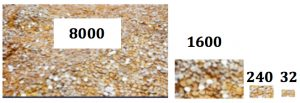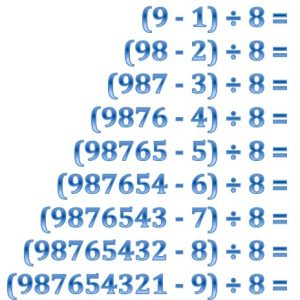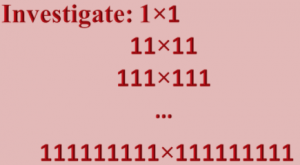What patterns do you see in them?

Suppose you have to share 9872 gold coins between 8 people.

You give the coins out in thousands until there are less than 8000 left.

Then you give out one hundred at a time until there are less than 800, then 10 at a time then 1 at a time.

You could do this sharing by splitting the coins into 4 piles and sharing each of the piles between the 8 people.

You give each person 1000 + 200 + 30 + 4 coins.Use the coin sharing story to explain the division sum:

9872 ÷ 8 = 1000 + 200 + 30 + 4 = 1234The brackets in these calculations tell you that you must do the calculation inside the brackets first.

Using the subtraction calculations that you have already done can you complete all of these calculations without using a calculator?

What patterns do you notice? Why do you think that the ancient Egyptians called these numbers magic numbers?As an ‘extra’, can you explain this pattern?